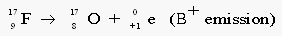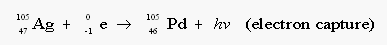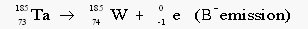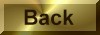Practice Problem 4

Predict the most likely modes of decay and the products of decay of the following nuclides:

(a)  17F     (b)  105Ag      (c)  185Ta

Answer

The first step in predicting the mode of decay of a nuclide is to decide whether the nuclide is neutron rich or neutron poor.  This can be done by comparing the mass number of the nuclide with the atomic weight of the element.

(a)  The atomic weight of flourine is 18.998 amu.  Because the mass of a  17F nuclide is smaller that the average fluorine atom, the 17F nuclide must contain fewer neutron.  It is therefore likely to be a neutron poor.   Because it is a relatively light nuclide,  17F  might be expected to decay by positron emission:(b)  The atomic weight of silver is 107.868 amu.  Because the mass of the 105Ag  nuclide is smaller than the average silver atom, this nuclide contains fewer neutrons than the stable isotopes of silver.  Since 105Ag  is a relatively heavy neutron-poor nuclide, we expect it to decay by electron capture:(c)  The atomic weight of tantalum is 180.948 amu.  The 185Ta isotope is therefore likely to be a neutron-rich isotope, which decays by elelctron emission: